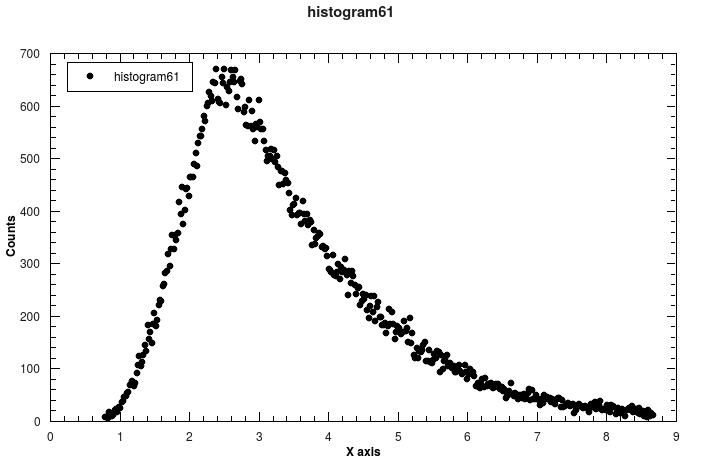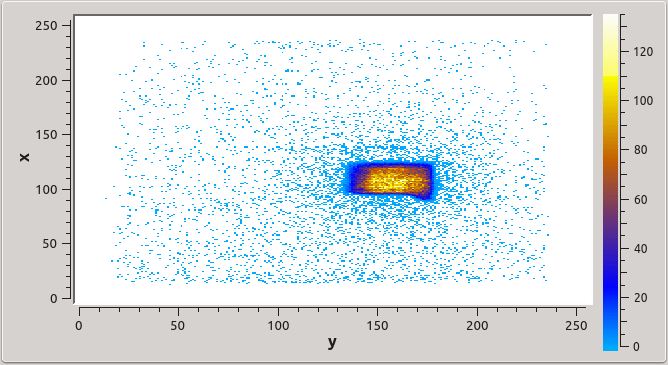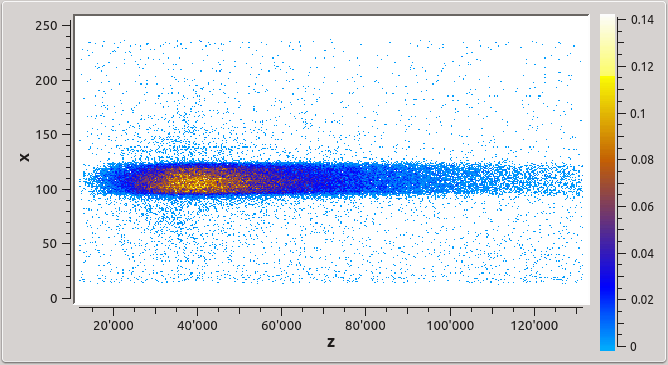$$\renewcommand\AA{\unicode{x212B}}$$

# ViewBOA v1¶

## Summary¶

Load a BOA file and create the 3 BOA plots.

## Properties¶

Name

Direction

Type

Default

Description

Year

Input

number

2023

Choose year

Numor

Input

number

0

Choose file number

CDDistance

Input

number

6

Chopper Detector distance in metres

## Description¶

The algorithm loads a BOA file using LoadSINQ v1. It produces three output workspaces, histogram N, ysum N and psdsum N, where N is the numor supplied to ViewBOA.

histogram N contains the wavelength spectrum and can be plotted using “Plot spectrum…”. The other two workspaces can be inspected with the spectrum slice viewer. psdsum N shows a beam profile on the two-dimensional detector, whereas ysum N shows the distribution of events with TOF on the x-axis and the detector x-coordinate on the y-axis, as indicated in the three images below (histogram, psdsum and ysum from left to right).## Usage¶

Note

The following code loads a BOA file and prints out information about the wavelength distribution of the neutrons.

import numpy as np

ViewBOA(2014, 61, 6)

print("The wavelength spectrum is described by {} points.".format(len(lamdba_distribution)))
print("The range covered is {0} - {1} Angstrom.".format(round(lambda_values, 4),round(lambda_values[-1], 4)))
print("The maximum is located at {} Angstrom.".format(round(lambda_values[np.argmax(lamdba_distribution)], 4)))


This script leads to the following output:

The wavelength spectrum is described by 400 points.
The range covered is 0.7912 - 8.6638 Angstrom.
The maximum is located at 2.3894 Angstrom.


In addition, the three workspaces described above are available, plotting them should produce figures like the above.

Categories: AlgorithmIndex | SINQ

## Source¶

Python: ViewBOA.py